International Journal of Biomedical Science and Engineering
Volume 4, Issue 1, February 2016, Pages: 1-6

Analysis by Simulation of the Factors Affecting the Amplitude and the Waveform of the Human Colon Slow Waves Recorded with Cutaneous Electrodes

Nicola Mirizzi1, *, Giuseppe Riezzo2, Paola DellAquila3

1Department of Physics, University of Bari, Bari, Italy

2Laboratory of Nutritional Pathophysiology IRCCS "S. de Bellis" National Institute for Digestive Diseases, Castellana Grotte (BA), Italy

3Department of Emergency, Policlinico, University of Bari, Bari, Italy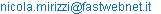(N. Mirizzi)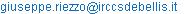(G. Riezzo)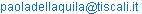(P. Dell’Aquila)

Nicola Mirizzi, Giuseppe Riezzo, Paola Dell'Aquila. Analysis by Simulation of the Factors Affecting the Amplitude and the Waveform of the Human Colon Slow Waves Recorded with Cutaneous Electrodes. International Journal of Biomedical Science and Engineering. Vol. 4, No. 1, 2016, pp. 1-6. doi: 10.11648/j.ijbse.20160401.11

Abstract: This study was undertaken to determine by simulation, the factors affecting the amplitude and the waveform of the human colon slow waves recorded with cutaneous electrodes. The study examined if the inter-electrode distance, the electrode positioning with respect to the projection of the longitudinal axis of the colon on the abdominal surface (technical factors) and of the thickness of the abdominal wall (anatomical factor) could have some effect on the peak amplitude and on the waveform of the cutaneous recordings. The simulation results indicated that there is an electrode configuration that optimizes the signal recorded with cutaneous electrodes and that the abdominal thickness has a profound effect on the slow wave amplitude due to the change in the distance between the source of the electrical signal (the colon) and the measuring electrodes. Moreover the simulation results indicated that the waveform of the cutaneous slow waves is complex with many peaks in each period and varies with the inter-electrodes distance.

Keywords: Computer Simulation, Extracellular Myoelectrical Activity, Human Colon, Cutaneous Electrodes

1. Introduction

The recording of the electrical activity of an internal organ from the abdominal surface is an interesting technique with possible clinical applications since it is not invasive, well-tolerated, and it allows long lasting recordings. The clinical application of such technique for diagnostic purposes requires to verify not only whether the recording of the cutaneous signal accurately reflects the electrical activity of the internal organ source of the recorded signal but also whether the changes in the features (amplitude, frequency, phase) of such signal are actually due to abnormalities in the physiology of the organ. The electrical signal recorded with cutaneous electrodes placed on the upper abdominal wall overlying the stomach (called Electrogastrography (EGG)) have underlined a good correlation with that recorded with serosal electrodes both in normal and in pathological conditions [1-4]. On the other side, the study of the cutaneous recordings of the electrical activity of the human colon has not had an equal development nor experimental nor by simulations. Perhaps this is due to the fact that the studies on the colonic slow waves recorded with implanted bipolar electrodes highlighted that their characteristics are highly irregular in amplitude, frequency and waveform and are time varying .

The modelling approach is useful to simulate the slow waves recorded with implanted bipolar electrodes, to analyze the factors affecting the amplitude and the waveform of the abdominal recordings, and to investigate the relationship between slow waves and EGG [9-11]. The dipole models to simulate the electrical activity of the human stomach [12-13] not only reproduces the electrical characteristics of the serosal slow waves and of spike bursts but also  indicates the optimal position of the cutaneous electrodes on the abdominal surface in accordance with the experimental results . The dipole model to simulate the electrical activity of the cat colon  not only reproduces the characteristics of the serosal slow waves and of spike bursts , but also analyzes the factors affecting the amplitude and the waveform of the serosal and of the cutaneous slow waves . The model to simulate the serosal electrical activity of the human colon  reproduces the electrical characteristics of the slow waves and indicates that their amplitude and frequency depend on electrophysiological factors (dipole moment value, annular band velocity), and on anatomical factors (geometrical size of the haustra).

On these bases, the aim of this study was to analyze, by simulation, the factors affecting the detectability of the human colon slow waves recorded with cutaneous electrodes since no previous study have yet investigated this subject. The cutaneous recordings of the electrical activity of the colon, like those of other organs, are a mixture of signal and noise due to the motion artifacts, the respiratory activity, the electrical activity from other organs, such as stomach, small and large intestine, heart.

To improve, at least in part, the detectability of the slow waves it is necessary to increase the signal-to-noise ratio by increasing their amplitude. For this reason, by simulation, we examined whether the inter-electrode distance, the electrode positioning with respect to the projection of the colon longitudinal axis on the abdominal surface (technical factors) and the different abdominal thickness (anatomical factor) could have some effect on the peak amplitude and on the waveform of the cutaneous recordings.

2. Material and Methods

2.1. The Basics of the Model

The simulation of the slow wave generation was based on the electric dipole model as reported in [12,13,15,18]. The ascending human colon was represented by a set of round ellipsoids of finite length in a Cartesian system of coordinates Oxyz (Figure 1a).

The plane Oxy represents the distal border of the colon segment, as reported in . The electrical potential V() generated by the d-wide annular band at the point P placed anywhere in the space near the cylinder was calculated by the following integral: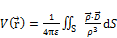(1)

whereis the vector distance between the point P located anywhere in the space near the cylinder and the origin O of the coordinates system Oxyz, e is the permittivity of the tissue where the electrodes were located, dS is an infinitesimal area segment of the d-wide annular band,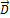is the vector dipole density of the annular band and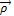is the vector distance between the point P and the infinitesimal area segment dS (Figure 1a).

Figure 1b shows the steps described below to represent the abdominal surface where the electrodes were positioned:

The abdominal surface was represented by means of a plane a.

The set of ellipsoids representing the colon was cut by means of a plane b parallel to the plane a. In our model the distance between the two planes represents the thickness of the abdominal wall. Since the media between the serosa of the human colon and the abdominal skin is mainly fat, the two plane were supposed separated by a dielectric of permittivity e = 2.213545E-4 C2/Nm2.

The axis z at the plane b was taken coincident with the longitudinal axis of the colon.

The cross-section of the set of ellipsoids lying in the plane b was projected on the plane a.

The system of coordinates O'x'y'z' was drawn parallel to the coordinate system Oxyz with the plane O'y'z' in the plane a. The x' axis is perpendicular to the plane a. The z' axis is the orthogonal projection of the longitudinal axis of the colon on the abdominal surface.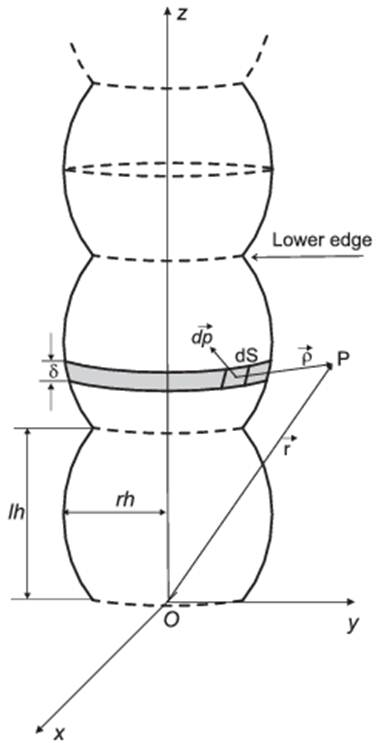a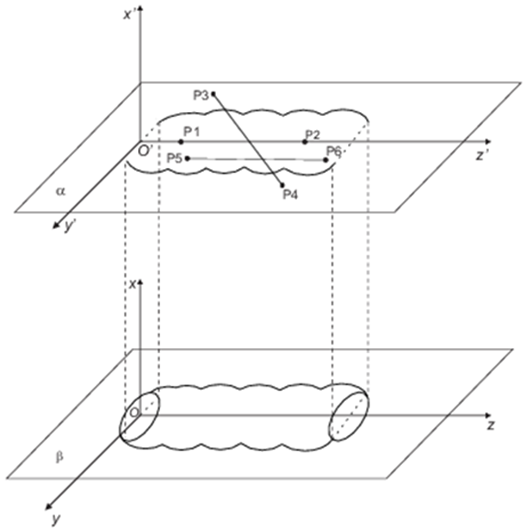b

Figure 1. Schematic representation of the colon. Figure 1a is the schematic representation of the ascending colon haustra in a Cartesian system of coordinate Oxyz; Figure 1b is the schematic representation of the colon haustra on the abdominal surface. The points P are the points where the electrodes were positioned.

2.2. Simulation Parameters

The simulations were performed by taking as reference the ellipsoid length lh = 0.05 m, the radius of the largest circle rh = 0.04 m, the dipole moment p = 1.1×10-8 Coulomb×m, the annular band velocity v = 0.0157 m/s corresponding to the frequency 4.7 cpm of the serosal simulated waveform , the inter-electrode distance taken as equal to 0.10 m.

In all the simulations one electrode was located at point P1 (see Figure 1b) at a distance of 0.06 m from the plane O’x’y’.

To simulate the effects of the thickness of the abdominal wall on the slow wave amplitude with effective data, the thickness was measured by ultrasonography on 14 subjects (4 female, 10 male) both in the supine position and in that lying on the side. The body mass index of the females ranged from 20.08 to 24.6 Kg/m2. The body mass index of the males ranged from 22.68 to 30.06 Kg/m2.

As reference for the thickness of the abdominal wall we took the value 0.013 m as measured from a female in the position lying on the side with BMI = 23.4 Kg/m2.

All simulations were performed on a laptop DELL in MATLAB.

3. Results and Discussion

3.1. Effects of the Inter-electrode Distance (Probe on the z’ Axis)

The analysis on such effects was performed by positioning the two electrodes on the z’ axis. One electrode was positioned at point P1 of coordinates P1(0.013, 0.0, 0.06) Such coordinates were not changed during the simulation. The coordinate z of the point P2 where the other electrode was placed was changed at the beginning of every cycle of simulation P2 (0.013, 0.0, i). The values 0.03, 0.05, 0.07, 0.09, 0.12 were assigned to i.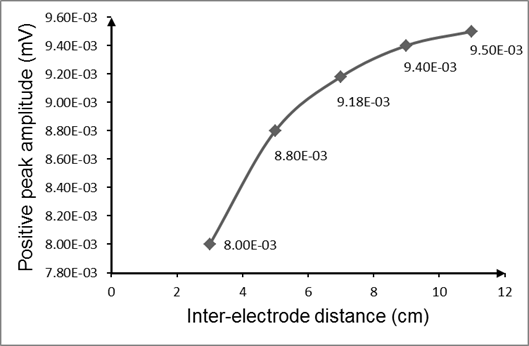Figure 2. Amplitude change of the positive peaks of the slow waves versus the inter-electrodes distance.

The simulation results are shown in Figure 2. It was found that the peak amplitude increases with the inter-electrode distance. For distance greater than 0.10 m the amplitude increment becomes small.

The results indicated also that the waveform of the cutaneous slow waves’ waveform is complex with many peaks in each period and varies with the inter-electrode distance (Figure 3a and Figure 3b).

We also checked whether the increase in the distance of the point P1 from the plane O’x’y’ could have any effect on peaks’ amplitude of the signal. The results shown in Table 1 point out that the increase in the distance of the point P1 from the plane O’x’y’ behaves increases in amplitude of the peak amplitude of the simulated slow waves.

Table 1. Positive peak amplitude changes related to increased distance of P1 from the plane O’x’y’.

 Parameters Values Coordinate z of P1 (m) 0.01 0.02 0.03 0.04 0.06 Positive peak amplitude (mV) 2.27E-03 2.68E-03 3.66E-03 6.52E-03 9.50E-03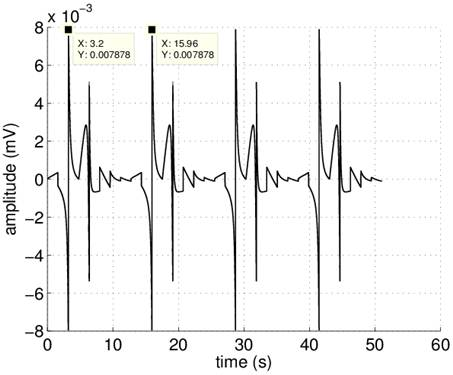Figure 3a. Simulated cutaneous slow waves. Simulated slow waves obtained with the electrodes on the axis z’ at points P1 of coordinates P1(0.013, 0.0, 0.006) and P2 of coordinates P2(0.013, 0.0. 0.09). The inter-electrode distance was 0.03 m; The peak amplitude of the wave form is 7. 87×10-3 mV.Figure 3b. Simulated cutaneous slow waves. Simulated slow waves obtained with the electrodes on the axis z’ at points P1 of coordinates P1(0.013, 0.0, 0.06) and P2 of coordinates P2((0.013, 0.0. 0.16) with the inter-electrode distance 0.10 m. The peak amplitude of the waveform is 9.47×10-3 mV. The period of both the waveforms is 12.76 s.

Besides the simulation pointed out that the increase in the distance of the point P1 from the plane O’x’y’ behaves also a significant modification of the waveform.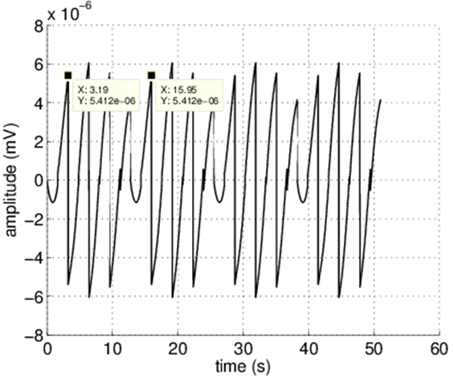Figure 4a. Effect of probe positioning respect to the z’ axis Slow wave obtained by placing the electrodes on the axis joining points P3 and P4 and forming an angle of 45° with the axis z’. The middle point of such axis was positioned at the distance 0.10 m from the plane O’x’y’. The peak amplitude of the wave form is 5.41×10-5 mV.

3.2. Effects of the Probe Positioning Respect to the z’ Axis

To investigate the effects of the electrode positioning with respect to the z’ axis the electrodes were placed at the points P3 of coordinates P3(0.013, -0.35, 0.065) and P4 of coordinates P4(0.013, 0.35, 0.135) (see Figure 1b) on an axis inclined 45° with respect to the axis z’. The distance between point P3 and P4 was taken equal to 0.10 m.

The simulation results shown in Figure 4a pointed out that the peak amplitude of the slow waves decreased when the angle between the axis z’ and the axis joining points P3 and P4 was increased. Besides the simulation pointed out that, when the probe was placed on an axis forming the angle 90° with the axis z’, the slow waves vanished.

To examine the effects of electrode placement on an axis parallel to axis z’, the electrodes were positioned in the plane a at points P5 of coordinates P5(0.013, 0.04, 0.06) and P6 of coordinates P6(0.013, 0.04, 0.16) (see Figure. 1b) The distance between the points P5 and P6 (inter-electrode distance) was equal to 0.10 m. P5 was at the distance 0.06 m from the plane O’x’y’. The simulation result in Figure 4b shows that the amplitude of the peaks is smaller than the amplitude obtained with electrodes on the axis z’ (see Figure 3a). Besides also the waveform is changed.

It follows that the amplitude depends on the orientation of the electrodes with respect to the axis z’. The positioning of the electrodes on an axis parallel to axis z’ modifies both the peak amplitude and the waveform also if they are placed on an axis whose distance from the axis z’ is small.

3.3. Effects of the Abdominal Wall Thickness

Since the electrical potential V() (1) generated by the annular band at the point P, depends on the vector distancebetween the point P and the infinitesimal area segment dS (Figure 1a), another factor that could influence the observability of the slow waves picked up with cutaneous electrodes is the thickness of the abdominal wall.

To investigate, by simulation, the influence of such factor we studied the effect of six measured abdominal wall thicknesses. The values of the parameters lh, rh, p, v, the inter-electrode distance and the distance of the point P1 from the plane O’x’y’ were taken equal to the reference values. The electrodes were placed on the axis z’.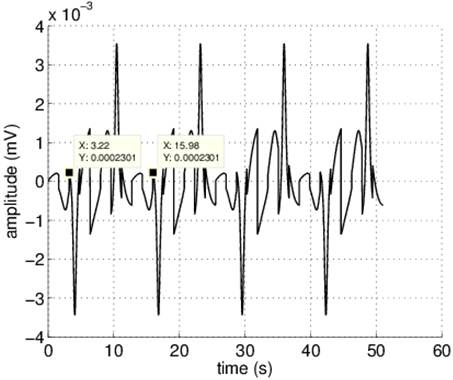Figure 4b. Effect of probe positioning with respect to the z’ axis. The electrode were placed at points P5 and P6 on an axis parallel to the axis z’ placed at a distance 0.04 m from the z’ axis. The peak amplitude of the wave form is 2.3×10-4 mV.

Table 2. Peak amplitude changes in cutaneous signal related to increased thickness of the abdominal wall.

 Parameters Values Abdominal thickness (m) 0.01 0.015 0.019 0.04 0.05 0.07 Positive peak amplitude (mV) 9.34 8.84 7.96 1.44 1.10 6.01 E-03 E-03 E-03 E-03 E-03 E-04

The results shown in Table 2 clearly indicated that thickness of the abdominal wall has a significant effect on the peaks’ amplitude of the slow waves recorded with abdominal electrodes. Such effect is due to the increase in the distance between the source of the electrical signal (the colon) and the measuring electrodes.

The simulated signals relative to the thickness 0.019 m and 0.07 m are shown in Figure 5a and Figure 5b, respectively. The simulation highlighted that also the waveform of the signal varies with the thickness of the abdominal wall. (Figure 5).

The simulation clearly revealed that the amplitude of the colon slow waves recorded with cutaneous electrodes depends at least on the following technical factors: the inter-electrodes distance, the electrode positioning with respect to the plane O’x’y’, the electrode position on the abdominal surface with respect to the longitudinal colon axis. Hence to optimize the detectability of the myoelectrical activity recorded with cutaneous electrodes it is highly recommended to localize the longitudinal axis of the tract of the colon under examination and the position of the right iliac fossa to determine the best location of electrodes.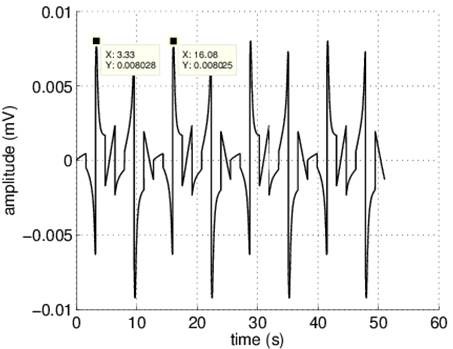Figure 5a. Effect of the abdominal wall thickness. The electrodes were positioned on the axis z’ at points P1 of coordinates P1(0.019, 0.0, 0.06) and P2 of coordinates P2(0.019, 0.0. 0.16). The thickness was taken equal to 0.019 m. The peak amplitude of the wave form is 8.02×10-3 mV.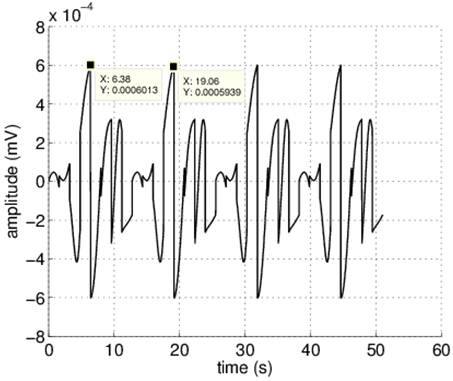Figure 5b. Effect of the abdominal wall thickness. The electrodes were positioned on the axis z’ at points P1 of coordinates P1(0.07, 0.0, 0.06) and P2 of coordinates P2(0.07, 0.0. 0.16). The thickness was taken equal to 0.07 m. The peak amplitude of the wave form is 6.01×10-4mV.

The simulation results indicate also that the slow wave amplitude is small and depends on the thickness of the abdominal wall (Table 2). The measurement of such thickness indicated that its value depends not only on the BMI but also on the subject position (supine or lying on the side).

Therefore, at the light of these findings, we think useful to determine experimentally the amplifier gain of the recording system and the subject position that improves the detection of the slow waves recorded with cutaneous electrodes. These results underline that it is useful to standardize the procedure to record the electrical activity of the colon from the abdominal surface.

We think that if the electrode placement and the subject position that optimize the slow wave amplitude are utilized and taken as standard, the cutaneous recordings can be better performed and more efficiently conducted and the amplitude becomes more reliable. However, in the experimental measurements, other factors not related to the electrode configuration and positioning such as the skin-electrode impedance and the dimension of the electrode leads, in the simulation considered as points, can affect the slow wave amplitude.

Moreover, the simulation results pointed out that the slow wave recorded from the abdominal surface has a complex shape with many peaks of different amplitude in each period. These peaks are due to the fact that every ellipsoid is source of its own signal with variable amplitude. From an electrical engineers’ perspective, the waveform of the cutaneous slow waves is the summation of the electrical potentials generated by the polarized annular band in every position with respect to the plane Oxy during its cyclic movement. The peak amplitude of the single pulse depends on the distance of the annular band from electrodes. While serosal recordings provide local information relative to the inter-electrode distance, the abdominal recordings present global information of the electrical activity of the tract of colon under examination.

4. Conclusion

Our findings suggest that the electrode positioning and configuration might have a substantial impact on the cutaneous observability of the colonic slow waves. Besides our study indicates that the variability of the abdominal wall separating the colon from the abdomen might be important when evaluating the reliability of the colonic slow waves recorded with skin electrodes from obese subjects. The next step will be to verify, by simulations, if it is possible to detect, with cutaneous electrodes, the modifications over the time both of the frequency and of the phase lag of the slow waves due to the geometrical configuration of the ellipsoids, of the frequency due to changes of the annular band and of the amplitude due to the dipole moment value. Successively, we will experimentally verify the effects on the slow waves amplitude due to the electrode configuration and positioning, to the subjects positioning (supine or lying on the side), and to the thickness of the abdominal wall.

Acknowledgments

The authors are grateful to Dr. Ing. Roberto Mirizzi for his valuable assistance in developing the simulation program.

References

1. M.P. Mintchev, Y.J. Kingma, and K.L. Bowes. "Accuracy of cutaneous recordings of gastric electrical activity", Gastroenterology, 104: 1273-1280, 1993.
2. F. Pezzolla, G. Riezzo, M.A. Maselli, and I. Giorgio."Electrical activity recorded from abdominal surface after gastrectomy or colectomy in humans", Gastroenterology, 97: 313-320, 1989.
3. G. Riezzo, F. Pezzolla, M.A Maselli, and I. Giorgio. "Electrical activity recorded from the abdominal surface before and after right hemicolectomy in man", Digestion, 55: 185-190, 1994.
4. G. Riezzo, F. Russo F, and F. Indrio. "Electrogastrography in adults and children: the strength, pitfalls, and clinical significance of the cutaneous recording of the gastric electrical activity", Biomed Research International, 282757, 2013.
5. S.K. Sarna, B.L. Bardakjian, W.E. Waterfall, J.F. Lind. "Human colonic electrical control activity (ECA)," Gastroenterology, 78: 1526-1536, 1980.
6. S.K. Sarna, W.E. Waterfall, B.L. Bardakjian, and J.F. Lind. "Types of human colonic electrical activities recorded postoperatively," Gastroenterology, 81: 61-70, 1981.
7. R.E. Condon, V.E. Cowles, A.A. Ferraz, S. Carilli, M.E. Carlson, K. Ludwig, E. Tekin, K. Ulualp, F. Ezberci, Y. Shoji, P. Isherwood, C.T. Frantzides, and W.J. Schulte. "Human colonic smooth muscle electrical activity during and after recovery from postoperative ileus," American Journal of Physiology, 269: G408-G417, 1995.
8. Taylor I, Duthie HL, Smallwood R, and Linkens D. "Large bowel myoelectrical activity in man," Gut, 16: 808-814, 1975.
9. S. Calder, LK Cheng, P Du. "A theoretical analysis of the electrogastrogram (EGG)". Annual International Conference of the IEEE Engineering in Medicine and Biology Society, 2014:4330-3, 2014.
10. P Du, G O'Grady, JB Davidson, LK Cheng, AJ Pullan. "Multiscale modeling of gastrointestinal electrophysiology and experimental validation", Critical Reviews in Biomedical Engineering, 38:225-54, 2010.
11. P Du, J Gao, G O'Grady, LK Cheng. "A simplified biophysical cell model for gastric slow wave entrainment simulation. Annual International Conference of the IEEE Engineering in Medicine and Biology Society, 2013:6547-50, 2013.
12. N. Mirizzi, R.Stella, and U. Scafoglieri."A model of extracellular waveshape of the gastric electrical activity," Medical Biological Engeenering & Computing, 23: 33-37, 1985.
13. N. Mirizzi, R. Stella, and U. Scafoglieri."Model to simulate the gastric electrical control and response activity on the stomach wall and on the abdominal surface," Medical & Biological Engineering & Computing, 24: 157-163, 1986.
14. N. Mirizzi and U. Scafoglieri."Optimal direction of the electrogastrographic signal in man." Medical & Biological Engineering & Computing, 21: 385–389, 1983.
15. N. Mirizzi, M.A. Strangio, R. Mirizzi, and G. Riezzo."Mathematical model to simulate the extracellular myoelectrical activity of the cat colon," Medical Engineering & Physics, 31: 145-152, 2009.
16. M. Wienbeck, "The electrical activity of the cat colon in vivo. I. The normal electrical activity and its relationship to contractile activity", Research in Experimental Medicine, 158: 268-79, 1972.
17. N.Mirizzi, R. Mirizzi, G. Riezzo."Computer simulation based on the electric dipole model: Analysis of the factor affecting the amplitude and the waveform of the cat colon electrical control activity," Journal of Medical and Biological Engineering, 30: 35-40, 2010.
18. N. Mirizzi, R. G. Riezzo."Analysis by Computer Simulation of Factors Affecting the Extracellular Slow Waves Features of the Human Colon" International. Journal of Biomedical Science and Engineering, 3: 18-24, 2015.

 Contents 1. 2. 2.1. 2.2. 3. 3.1. 3.2. 3.3. 4.
Article ToolsAbstractPDF(2148K)